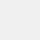# Camera Math - Homework Hel‪p

نسخهٔ ۱.۳.۸
 نصب +۱ هزار از ۳ رأی ۲.۳ دسته‌بندی آموزش حجم ۵۸ مگابایت آخرین بروزرسانی ۴ مهر ۱۴۰۲# Camera Math - Homework Hel‪p

Crazysloth Pte. Ltd
نسخهٔ ۱.۳.۸
 نصب +۱ هزار از ۳ رأی ۲.۳ دسته‌بندی آموزش حجم ۵۸ مگابایت آخرین بروزرسانی ۴ مهر ۱۴۰۲
مشاهده‌ی نتایج بررسی آنتی‌ویروس

## معرفی برنامه

جزئیات بیشتر

Attention students! Camera Math solves all your math problems. Yes, by all we mean word problems too. Simply take a photo of the question and Camera Math will automatically find the best solution to it.

Camera Math is an essential learning and problem-solving tool for students! Just snap a picture of the question of the homework and Camera Math will show you the step-by-step solution with detailed explanations.
Camera Math covers all levels of math including Arithmetic, Algebras, Trigonometry, Pre-Calculus, Calculus, and more.
【Features】
- Arithmetic: addition, subtraction, multiplication, division, fractions, decimals
- Algebra I: systems of equations, functions, real numbers, exponents, rational expressions
- Algebra II: linear equations, inequalities, graphs, matrices, polynomials and radical expressions, quadratic equations
- Pre-calculus: functions and graphs, lines and rates of change, sequences and series, polynomial and rational functions, exponential and logarithmic functions, analytic geometry
- Calculus: limits, derivatives, integrals
- Trigonometric: sine function, cosine function and tangent function
- Statistics: analyzing categorical data, displaying and comparing quantitative data, summarizing quantitative data
- Geometry: points, lines, planes, angles, parallel lines, triangles, similarity, trigonometry, quadrilaterals, transformations, circles and area

【Description】
Camera Math is a simple and efficient tool application for math problem solvers. Take a photo and search questions without any ads to get answers, and learn more efficiently for you.
Don’t know answers to the math questions, and no one around to help with math homework? Camera Math is your personal assistant for math homework. When you find a math problem that you don’t know how to solve, you can easily take a photo with Camera Math, and you’ll get the answer in a few seconds. Camera Math provides you with detailed explanations of the questions to help you learn math effortlessly.
At the same time, welcome to join our community group to talk with more people, provide us with feedback, ask for help from others, open to discuss with more topics and meet more new friends.
- Take a photo for your math problem in a snap, easy to use and efficient.
- Recognize math problems quickly and accurately. The homework solver is like a teacher on the spot.
- Cover diverse mathematical problems for students at all levels, whether it is an expression or a text problem.
- The whole network search provides diversified problem-solving methods, and provides you with at least two options for the same problem.
- Get detailed step-by-step solutions for solving math problems to deepen your understanding of math problems, help you deeply understand where you are wrong in order to improve math performance.
- The scientific calculator can support manual editing of mathematical questions in the math keyboard.
- Keep historical search records, review them at any time.
- Without any ads to get answers more efficiently, and without any charge.
Instagram : https://www.instagram.com/cameramath/

نظرات کاربران - ۳ رأی
۲.۳ از ۵
۵
۴
۳
۲
۱دانیال
۱۴۰۱/۰۹/۲۱
اصلا خوب نیست کار نمیکنهشاهو
۱۴۰۱/۰۲/۳۱
خیلی بده نصب نکنیدمجید ش
۱۴۰۰/۱۱/۲۷
خیلی عالیه پیشنهاد میکنم حتما نصب کنید ♥︎♥︎